Mayan Numeral Exercises Write the following Mayan numerals in decimal notation (base 10). `1.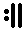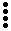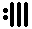2.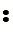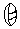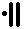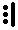3.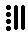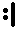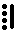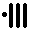`  Add the following (without converting to decimal form). ```4.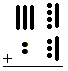5.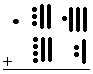6.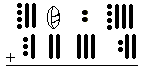```  Subtract the following (without converting to decimal form). `7.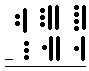8.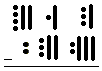9.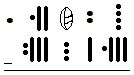`  Write the following in Mayan numerals.`10. 238 11. 1280 12. 239 836` Exercise Answers Back to Mayan Numerals main page. To return to the previous page use your browser's back button.